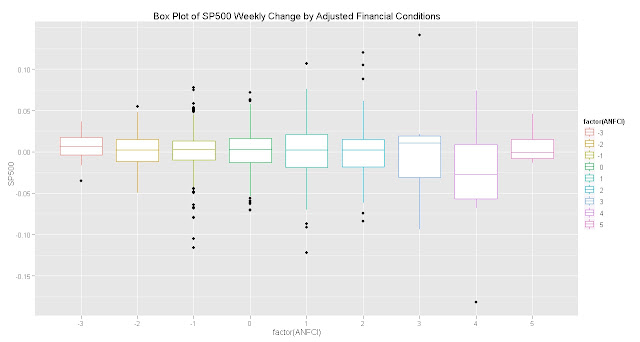Want to share your content on R-bloggers? click here if you have a blog, or here if you don't.

I owe someone at the Fed a beer for all the recent additions at http://research.stlouisfed.org/fred2/.  I have covered some in Spreads and Stress and Gifts from BAC ML and the Federal Reserve.  The newest addition 8 Chicago Fed Indexes Added to FRED contains a nice weekly series on US National Financial Conditions that I’m sure we can use.

How does the S&P 500 look against the Chicago Fed Adjusted National Financial Conditions (ANFCI) index?  I did not look at the nonadjusted FCI, but I’m sure it offers potentially useful information also.From TimelyPortfolio

Can we build a silly system with the S&P 500 and ANFCI?  THIS IS MERELY AN ILLUSTRATION AND DOES NOT CONSTITUTE FINANCIAL ADVICE.  YOUR GAINS AND LOSSES ARE YOUR OWN RESPONSIBILITY.

Nothing particularly special system-wise, but 1998-2004 is a very abnormal circumstance.

R code:

```#explore how SP500 behaves in different ranges of Financial Conditions   require(quantmod)
require(PerformanceAnalytics)
require(ggplot2)   #get data from St. Louis Federal Reserve (FRED)
chartSeries(ANFCI,theme="white",name="Adjusted National Financial Conditions (ANFCI)")   #do a little manipulation to get the data lined up on weekly basis
SP500  <-  to.weekly(SP500)[,4]
ANFCI <- to.weekly(ANFCI)[,4]
#get weekly format to yyyy-mm-dd with the first day of the week
index(SP500) <- as.Date(index(SP500))
index(ANFCI) <- as.Date(index(ANFCI))   #use ceiling to get ranges for ANFCI so we can run boxlplots
ANFCI <- floor(ANFCI)
#lag ANFCI signal
ANFCI <- lag(ANFCI,k=1)   #merge sp500 returns and ANFCI
SP500_ANFCI <- na.omit(merge(ROC(SP500,n=1,type="discrete"),ANFCI))
colnames(SP500_ANFCI) <- c("SP500","ANFCI")   #convert xts to data frame for ggplot boxplot exploration
df <- as.data.frame(cbind(index(SP500_ANFCI),
coredata(SP500_ANFCI[,1:2])))
ggplot(df,aes(factor(ANFCI),SP500)) +
geom_boxplot(aes(colour = factor(ANFCI))) +
opts(title="Box Plot of SP500 Weekly Change by Adjusted Financial Conditions")       #show linked returns based on Adjusted Financial Conditions
ret_eq_neg3 <- ifelse(SP500_ANFCI[,2] == -3, SP500_ANFCI[,1], 0)
ret_eq_neg2 <- ifelse(SP500_ANFCI[,2] == -2, SP500_ANFCI[,1], 0)
ret_eq_neg1 <- ifelse(SP500_ANFCI[,2] == -1, SP500_ANFCI[,1], 0)
ret_eq_0 <- ifelse(SP500_ANFCI[,2] == 0, SP500_ANFCI[,1], 0)
ret_eq_pos1 <- ifelse(SP500_ANFCI[,2] == 1, SP500_ANFCI[,1], 0)
ret_eq_pos2 <- ifelse(SP500_ANFCI[,2] == 2, SP500_ANFCI[,1], 0)
ret_eq_pos3 <- ifelse(SP500_ANFCI[,2] == 3, SP500_ANFCI[,1], 0)
ret_eq_pos4 <- ifelse(SP500_ANFCI[,2] == 4, SP500_ANFCI[,1], 0)
ret_eq_pos5 <- ifelse(SP500_ANFCI[,2] == 5, SP500_ANFCI[,1], 0)   #merge series for PerformanceSummary chart
ret_comp <- merge(ret_eq_neg3, ret_eq_neg2, ret_eq_neg1, ret_eq_0,
ret_eq_pos1,ret_eq_pos2,ret_eq_pos3,ret_eq_pos4,ret_eq_pos5)
#name columns for the legend
colnames(ret_comp) <- c("ANFCI=-3","ANFCI=-2","ANFCI=-1","ANFCI=0",
"ANFCI=1","ANFCI=2","ANFCI=3","ANFCI=4","ANFCI=5")   charts.PerformanceSummary(ret_comp, main="SP500 Linked Returns by Financial Conditions")       #and just for fun a very basic system
signal <- runMax(SP500_ANFCI[,2] , n=10)
#go long if Max of ANFCI over last ten weeks is 0; already lagged earlier
ret <- ifelse(signal <= 0, 1, 0) * SP500_ANFCI[,1]
ret <- merge(ret, SP500_ANFCI[,1])
colnames(ret) <- c("ANFCI_LongOnlySystem","SP500")
charts.PerformanceSummary(ret, ylog=TRUE, main="Very Simple ANFCI S&P 500 System",